# Finding the Area of compound shapes (all 2D shapes)

In this worksheet, students will use the areas of Rectangles, Triangles, Parallelograms and Trapeziums to find the Area of compound shapes.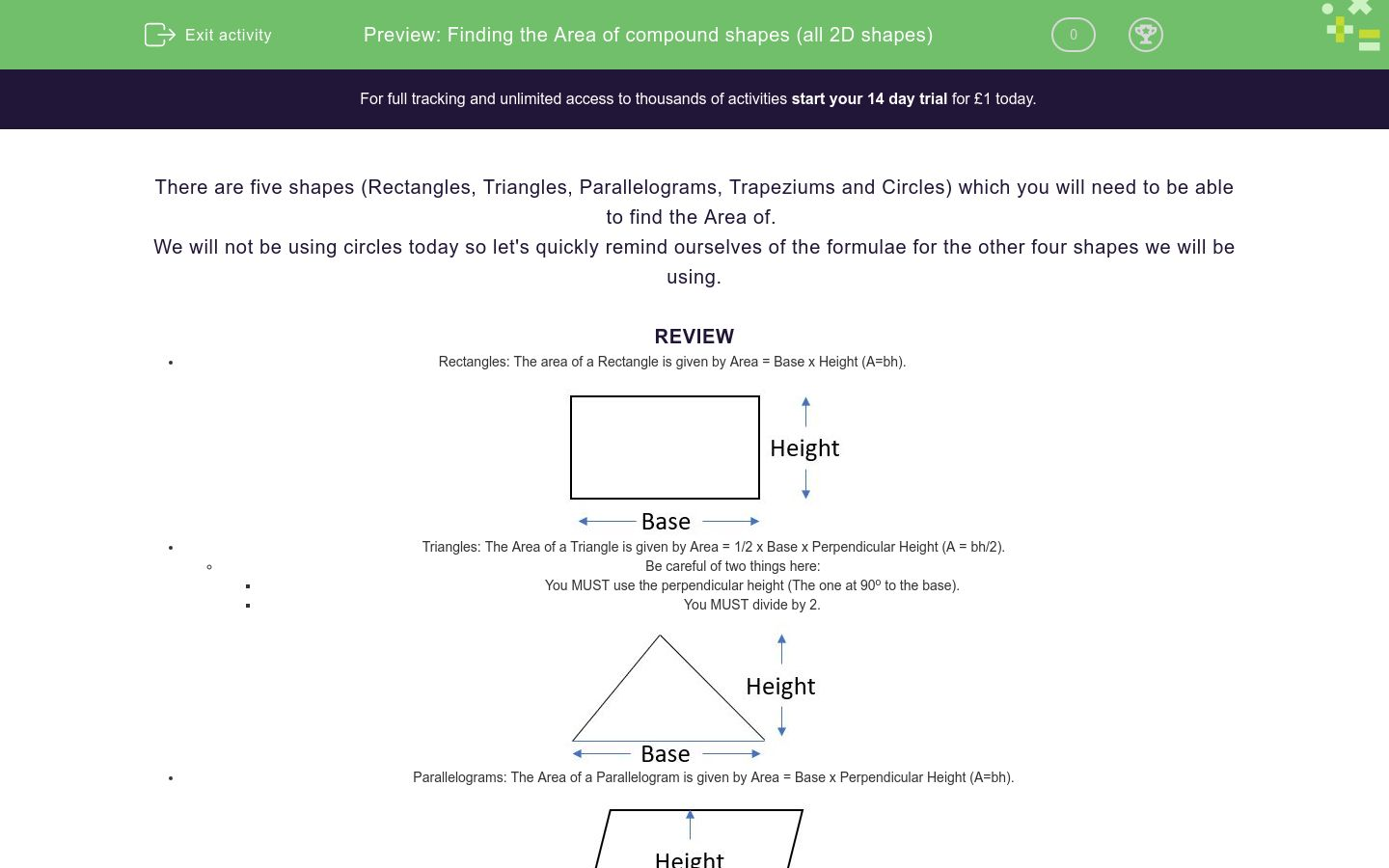Key stage:  KS 3

Curriculum topic:   Geometry and Measures

Curriculum subtopic:   Solve Problems Involving Perimeters and Areas of 2D Shapes

Difficulty level:### QUESTION 1 of 10

There are five shapes (Rectangles, Triangles, Parallelograms, Trapeziums and Circles) which you will need to be able to find the Area of.

We will not be using circles today so let's quickly remind ourselves of the formulae for the other four shapes we will be using.

REVIEW

• Rectangles: The area of a Rectangle is given by Area = Base x Height (A=bh).• Triangles: The Area of a Triangle is given by Area = 1/2 x Base x Perpendicular Height (A = bh/2).
• Be careful of two things here:
• You MUST use the perpendicular height (The one at 90o to the base).
• You MUST divide by 2.• Parallelograms: The Area of a Parallelogram is given by Area = Base x Perpendicular Height (A=bh).• Trapeziums. The area of a Trapezium is given by Area = 1/2 x Sum of the Parallel Sides x Perpendicular Height (1/2 x (a + b) x h).
• Be careful of two things here:
• You must divide by 2.
• a and b are the parallel sides, remember that they could be the sides instead of the top and bottom.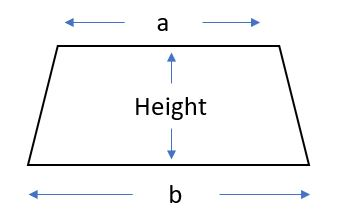EXAMPLE: Find the Area of this compound shape.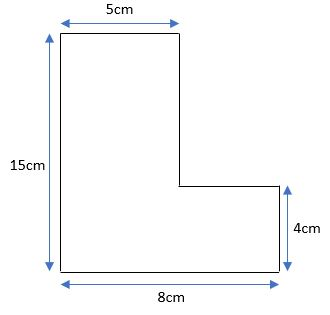• Split the shape into two or more shapes that we can deal with. In this case, it splits nicely into two rectangles.
• Work out the lengths we are not given. We may not need them, but it's useful to have them in case we do.
• Label the shapes separately so you know which one you are talking about in your working.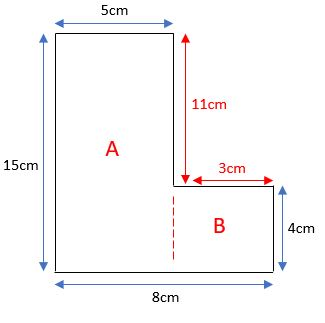• The base of A is 5cm and the height of A is 15cm. The Area of A is 75cm2.
• The base of B is 3cm and the height of B is 4cm. The Area of B is 12cm2.
• The total Area of the shape is 75cm2 + 12cm2 = 87cm2.

In this worksheet, use your knowledge to calculate the Area of each shape.

Find the Area of this compound shape;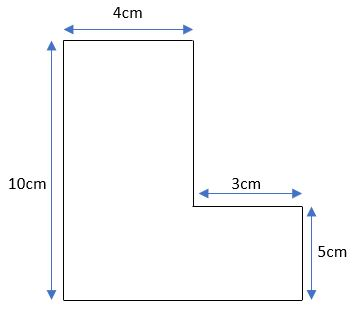55

600

50

Find the Area of this compound shape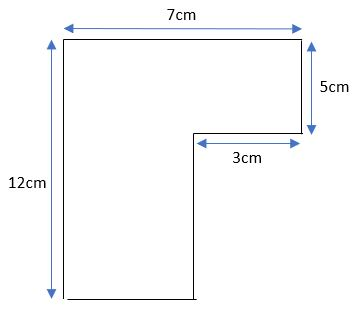99

63

720

Find the Area of this compound shape82

58

49

Find the Area of this compound shape45

50

35

Find the Area of the following compound shape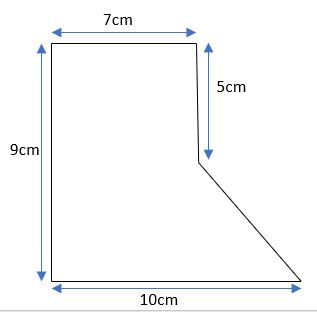75

70.5

69

Find the Area of this compound shape.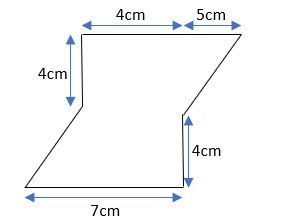64

48

560

Find the Area of this compound shape.45

55

115

Find the Area of this compound shape.84

57

48

Find the Area of this compound shape.182

142

106

Find the Area of the given compound shape.77

84

90

• Question 1

Find the Area of this compound shape;55
EDDIE SAYS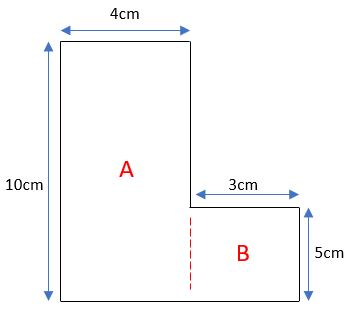The Area of this shape can be found by splitting the shape into two rectangles as follows: The Area of A is (4 x 10)= 40cm2 The Area of B is (3 x 5) = 15cm2 The total Area is 55cm2
• Question 2

Find the Area of this compound shape63
EDDIE SAYSThe Area of this shape can be found by splitting the shape into two rectangles as follows: We need to find the base of shape A to find the area, this will be 4cm. The Area of Shape A = (12 x 4) = 48cm2 The Area of Shape B = (3 x 5) = 15cm2 The total Area of the shape = (48 + 15) = 63cm2
• Question 3

Find the Area of this compound shape58
EDDIE SAYSThe Area of this shape can be found by splitting the shape into a rectangle and two squares as follows: The Area of Shape A = (10 x 4) = 40cm2 The Area of Shape B = (3 x 3) = 9cm2 The Area of Shape C = (3 x 3) = 9cm2 The total Area of the shape = (40 + 9 + 9) = 58cm2
• Question 4

Find the Area of this compound shape35
EDDIE SAYSThe Area of this shape can be found by splitting the shape into a rectangle and right angled triangle as follows: The Area of shape A = (4 x 5) = 20m2 The Area of shape B = (6 x 5) / 2 = 15m2 The total Area is 20 + 15 = 35m2
• Question 5

Find the Area of the following compound shape69
EDDIE SAYSThe Area of this shape can be found by splitting the shape into a rectangle and right angled triangle as follows: The Area of shape A is (7 x 9) = 63cm2 The Area of shape B is (3 x 4) / 2 = 6cm2 The total Area is 63 + 6 = 69cm2
• Question 6

Find the Area of this compound shape.48
EDDIE SAYSThis one is a bit harder to spot. We have to split it into three shapes, a rectangle and two triangles. Note that the triangles have different bases so we have to find their areas separately. The Area of shape A is (3 x 4) / 2 = 6cm2 The Area of shape B is (8 x 4) = 32cm2 The Area of shape A is (5 x 4) / 2 = 10cm2 The total Area is 6 + 32 + 10 = 48cm2
• Question 7

Find the Area of this compound shape.45
EDDIE SAYSThe Area of this shape can be found by splitting the shape into a rectangle and trapezium as follows: Shape A is a rectangle so Area = (5 x 3) = 15 Shape B is a trapezium so Area = (10 + 5) x 4 / 2 = 30 The total Area is 15 + 30 = 45cm2
• Question 8

Find the Area of this compound shape.57
EDDIE SAYSThe Area of this shape can be found by splitting the shape into a rectangle and trapezium as follows: Shape A is a rectangle so Area = (6 x 5) = 30 Shape B is a trapezium so Area = (6 + 3) x 6 / 2 = 27 The total Area of the shape is 30 + 27 = 57cm2
• Question 9

Find the Area of this compound shape.106
EDDIE SAYSThe Area of this shape can be found by splitting the shape into a parallelogram and trapezium as follows: Shape A is a parallelogram so Area = (5 x 6) = 30 Shape B is a trapezium so Area = (5 + 14) x 8 / 2 = 76 The total Area of the shape is 30 + 76 = 1062
• Question 10

Find the Area of the given compound shape.77
EDDIE SAYSFor this question, you need to notice the litte dashes on the sides, this means that these sides are all the same. Shape A is a square so Area = (7 x 7) = 49 Shape B is a parallelogram so Area = (7 x 3) = 21 Shape C is a triangle so Area = (7x 2) / 2 = 7 The total Area is 49 + 21 + 7 = 77cm2
---- OR ----

Sign up for a £1 trial so you can track and measure your child's progress on this activity.

### What is EdPlace?

We're your National Curriculum aligned online education content provider helping each child succeed in English, maths and science from year 1 to GCSE. With an EdPlace account you’ll be able to track and measure progress, helping each child achieve their best. We build confidence and attainment by personalising each child’s learning at a level that suits them.

Get started